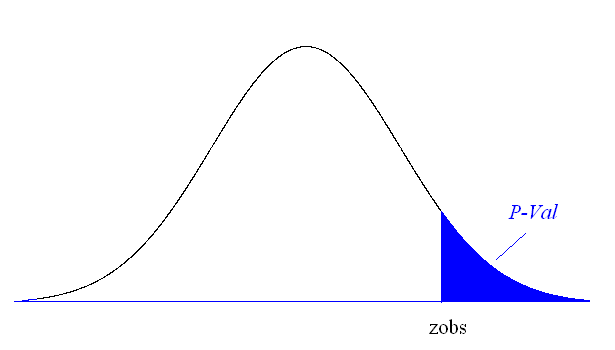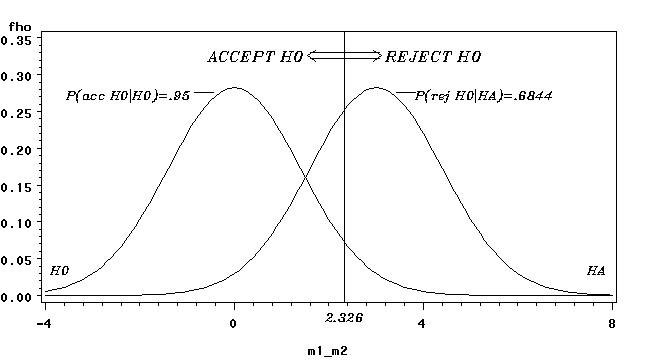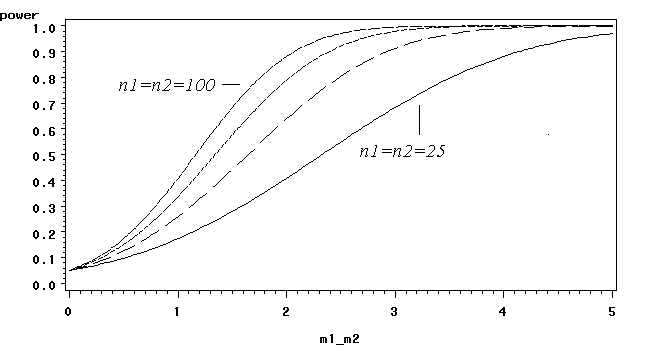## Hypothesis testing

• Goal: Make statement(s) regarding unknown population – Null hypothesis - Statement regarding the value(s) of unknown
parameter(s). Typically will imply no association between explanatory and response variables in our applications (will always contain an equality) – Alternative hypothesis - Statement contradictory to the null
hypothesis (will always contain an inequality) – Test statistic - Quantity based on sample data and null
hypothesis used to test between null and alternative hypotheses – Rejection region - Values of the test statistic for which we
reject the null in favor of the alternative hypothesis α = P(Type I Error) β = P(Type II Error) • Goal: Keep α, β reasonably small • Drug company has new drug, wishes to compare it • Federal regulators tell company that they must demonstrate that new drug is better than current treatment to receive approval • Firm runs clinical trial where some patients receive new drug, and others receive standard treatment • Numeric response of therapeutic effect is obtained • Null hypothesis - New drug is no better than standard trt
Alternative hypothesis - New drug is better than standard trt
Experimental (Sample) data:
Sampling Distribution of Difference in Means • In large samples, the difference in two sample means is • Under the null hypothesis, μ -μ =0 and: • σ 2 and σ 2 are unknown and estimated by s 2 and s 2 • Type I error - Concluding that the new drug is better than the
standard (H ) when in fact it is no better (H ). Ineffective drug is – Traditionally α = P(Type I error) = 0.05 • Type II error - Failing to conclude that the new drug is better
(H ) when in fact it is. Effective drug is deemed to be no better.
– Traditionally a clinically important difference (Δ) is assigned β = P(Type II error | μ -μ = Δ) ≤ .20 • Test Statistic - Difference between the Sample means,
scaled to number of standard deviations (standard errors)
from the null difference of 0 for the Population means:
Rejection Region - Set of values of the test statistic that are
consistent with H , such that the probability it falls in this
region when H is true is α (we will always set α=0.05)P-value (aka Observed Significance Level) • P-value - Measure of the strength of evidence the sample
data provides against the null hypothesis: P(Evidence This strong or stronger against H | H is true) P val : p = P(Z z Large-Sample Test H :μ -μ =0 vs H :μ -μ >0 • H : μ -μ = 0 (No difference in population means
H : μ -μ > 0 (Population Mean 1 > Pop Mean 2)
P value : P ( Z zConclusion - Reject H if test statistic falls in rejection region,
or equivalently the P-value is ≤ α • Patients - Individuals suffering from cervical dystonia
Response - Tsui score of severity of cervical dystonia
(higher scores are more severe) at week 8 of Tx • Research (alternative) hypothesis - Botox A
decreases mean Tsui score more than placebo • Groups - Placebo (Group 1) and Botox A (Group 2)
Experimental (Sample) Results:
Test whether Botox A produces lower mean Tsui scores than placebo (α = 0.05) • P val : P(Z ≥ 2. ) Conclusion: Botox A produces lower mean Tsui scores than
placebo (since 2.82 > 1.645 and P-value < 0.05)
• Many studies don’t assume a direction wrt the • H : μ -μ = 0 H : μ -μ ≠ 0 – Do not reject μ -μ = 0 if -zP-value: 2P(Z|zPower - Probability a test rejects H (depends on μ - μ )
H True: Power = P(Type I error) = α – H False: Power = 1-P(Type II error) = 1-β · Example:
· H : μ - μ = 0 H : μ - μ > 0 · Decision Rule: Reject H (at α=0.05 significance level) if: • Now suppose in reality that μ -μ = 3.0 (H is true) • Power now refers to the probability we (correctly) reject the null hypothesis. Note that the sampling distribution of the difference in sample means is approximately normal, with mean 3.0 and standard deviation (standard error) 1.414.
• Decision Rule (from last slide): Conclude population means differ if the sample mean for group 1 is at least 2.326 higher than the sample mean for group 2 • Power for this case can be computed as: • As sample sizes increase, power increases • As population variances decrease, power increases • As the true mean difference increases, power increasesPower Curves for group sample sizes of 25,50,75,100 and varying true values μ -μ with σ =σ =5.
• For given μ -μ , power increases with sample size • For given sample size, power increases with μ -μ • Goal - Choose sample sizes to have a favorable chance of
detecting a clinically meaning differenceStep 1 - Define an important difference in means:
Case 1: σ approximated from prior experience or pilot study - dfference
Case 2: σ unknown - difference must be stated in units of standard
Step 2 - Choose the desired power to detect the the clinically
meaningful difference (1-β, typically at least .80). For 2-sided test:
Trts - Rosiglitazone vs Placebo
Response - Change in Limb fat mass
Clinically Meaningful Difference - 0.5 (std dev’s)
Desired Power - 1-β = 0.80
Significance Level - α = 0.05
• Normally Distributed data - approximately 95% of individual measurements lie within 2 standard deviations of the mean • Difference between 2 sample means is approximately normally distributed in large samples (regardless of shape of distribution of individual measurements): • Thus, we can expect (with 95% confidence) that our sample mean difference lies within 2 standard errors of the true difference (1-α)100% Confidence Interval for μ -μ • Large sample Confidence Interval for μ -μ : • Standard level of confidence is 95% (z • (1-α)100% CI’s and 2-sided tests reach the same conclusions regarding whether μ -μ = 0 • Comparison of Viagra (Group 1) and Placebo (Group 2) • Data pooled from 6 double-blind trials • Response - Percent of succesful intercourse attempts in past 4 weeks (Each subject reports his own percentage)